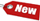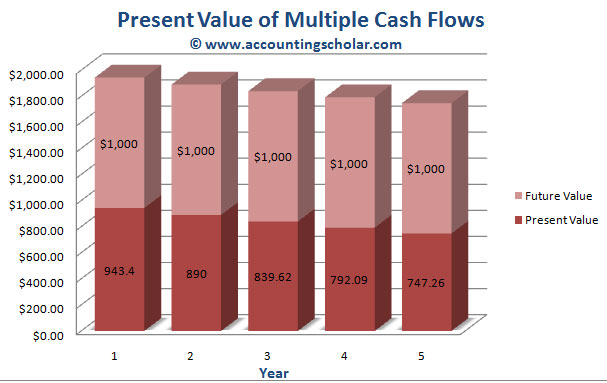Browse Accounting Lessons HereAccounting Terms & DefinitionsAccounting for Merchandising ActivitiesDebits and Credits (Double Entry Accounting)Business Valuation FormulasTime Value of Money & Present/Future ValuesComplex Debt & Equity InstrumentsCommon Stock & Shareholder's EquityAccounting & Finance RatiosValuing Common StockCorporate Income TaxesLower of Cost or Market (LCM) & Inventory ValuationChart of Accounts & BookkeepingBonds Payable & Long Term LiabilitiesCapital AssetsGAAP, Accrual & Cash Accounting, Information Commodity, Internal Controls & MaterialityWhat category of browser are you on this website? Accounting student (homework help) Finance professor (university research) Accounting manager (at work) Other Explore Careers in Accounting and FinanceVisit our section on Careers in Accounting & Finance to explore vast opportunities in this industry.

Chapter 4.12® - Compound the Accumulated Balance Forward One Year at a Time - Discounted Cash Flow Valuation - Determining Present Value of Multiple Future Cash Flows & Designing a Financial Timeline

To illustrate this concept of compounding the accumulated balance forward one year at a time, consider \$2000 invested at the end of each year of the next five years. The current balance is 0, that’s why on this timeline, we indicate Time 0 as \$0. The rate of return is 10%.

 0_________ \$0 1_________ \$2,000 2_________ \$2,000 3_________ \$2,000 4_________ \$2,000 5_________ \$2,000 > Time (years) > Cash Flows

 0_________ Bgn. Amount 1_________ \$0 2_________ \$2,200 3_________ \$4,620 4_________ \$7,282 5_________ \$10,220.20 > Time (years) > Cash Flows + Additions +\$2,000 +\$2,000 +\$2,000 +\$2,000 +\$2,000 End. Amount \$2,000 x 1.1 \$4,200 x 1.1 \$6,620 x 1.1 \$9,282 x 1.1 \$12,210.20

Notice nothing happens till the end of Year 1 (marked as Time 1 on the timeline) when we make the \$2000 investment. This first \$2,000 earns interest for the next four years (and not five years because we are contributing at the end of the year, as opposed to beginning of the year). Also notice that the last \$2,000 is invested at the end of the fifth year, so it earns no interest at all. Thus at the end of the fifth year, we have a final answer of \$12,210.20, that is the future value of all these present cash flows made through the years. Below is a graphical representation of the growth of this \$2000 in each of the years for five years.This graph above shows the growth of \$2,000 invested at the end of each year for the next five years at a rate of 10% return. The original investment of \$2,000 per year is shown in pink while the beginning balance of each year in shown in green & the ending balance each year is shown in purple.

Determining Present Value of Multiple Future Cash Flows

In the discount cash flow valuation of future cash flows, we looked at what could we have in 2 years if we invest \$100 now and \$100 in one year. In this tutorial, we look at what we need to determine the present value of a series of future cash flows, thus how much do we need now to have a set future value, of say \$3000 in 2 years. The context is therefore slightly changed; we are looking at cash flows valuation from a present perspective.

As an example, suppose you need \$1000 in one year, and another \$2000 more in two years. If you can earn 8% on your investments, how much do you have to put up today to exactly cover these amounts in the future? In paraphrased terms, what is the present value of the two cash flows at 8%?

The present value of \$2,000 in two years at 8% is (using the present value discount factor):

 Present Value = \$1 x [1/ (1 + r) t] = \$1/ (1 + r) t \$2000 / 1.082 \$2000 / 1.1664 = \$1714.68

The present value of \$1,000 in one year at 8% is (using the present value discount factor):

 Present Value = \$1 x [1/ (1 + r) t] = \$1/ (1 + r) t \$1000 / 1.081 \$925.93

As an example, suppose we had an investment that is going to pay \$1,000 at the end of every year for the next five years. To find the present value, we could discount each \$1000 in the five years to the present value separately and then add them up. Here is how we could do this in a financial timeline:

 0_________ 1_________ \$1,0000 2_________ \$1,000 3_________ \$1,000 4_________ \$1,000 5_________ \$1,000 > Time (years) > Cash Flows ___\$943.40__ x 1 / 1.06 ___\$890___ x 1 / 1.062 __\$839.62___ x 1 / 1.063 __\$792.09___ x 1 / 1.064 __\$747.26___ x 1 / 1.065 \$4212.37 = Total Present Value at 6%

Note the final answer we derive is \$4212.37. This number simply means that if we have an investment that pays \$1000 per year for the next five years at a rate of 6%, then the present value for this investment is \$4212.37. Note that if we were to increase the interest rate to say 12%, then this number will have a higher value, and will be higher. Below is a graphical representation of this investment comparing its present values & future values per each year:This graph above shows the present value of \$1,000 for each of the next five years with the amount being reduced each year. For instance, the present value of \$1,000 in the first year @ 12% rate of return is \$943.40. If we do the present value calculation for each of the 5 years, we would end up with a final present value answer of \$4,212.37.